Open In App
Related Articles

# QuickSort – Data Structure and Algorithm Tutorials

QuickSort is a sorting algorithm based on the Divide and Conquer algorithm that picks an element as a pivot and partitions the given array around the picked pivot by placing the pivot in its correct position in the sorted array.

## How does QuickSort work?

The key process in quickSort is a partition(). The target of partitions is to place the pivot (any element can be chosen to be a pivot) at its correct position in the sorted array and put all smaller elements to the left of the pivot, and all greater elements to the right of the pivot.

Partition is done recursively on each side of the pivot after the pivot is placed in its correct position and this finally sorts the array.How Quicksort works

Recommended Practice

### Choice of Pivot:

There are many different choices for picking pivots.

### Partition Algorithm:

The logic is simple, we start from the leftmost element and keep track of the index of smaller (or equal) elements as i. While traversing, if we find a smaller element, we swap the current element with arr[i]. Otherwise, we ignore the current element.

Let us understand the working of partition and the Quick Sort algorithm with the help of the following example:

Consider: arr[] = {10, 80, 30, 90, 40}.

• Compare 10 with the pivot and as it is less than pivot arrange it accrodingly.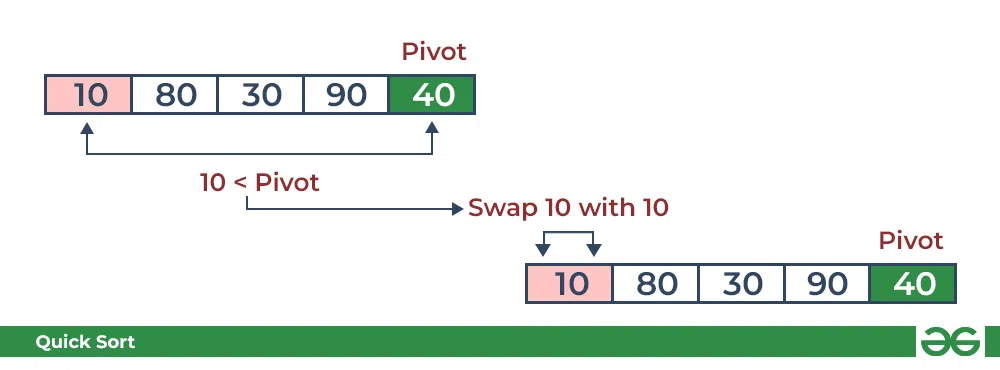Partition in QuickSort: Compare pivot with 10

• Compare 80 with the pivot. It is greater than pivot.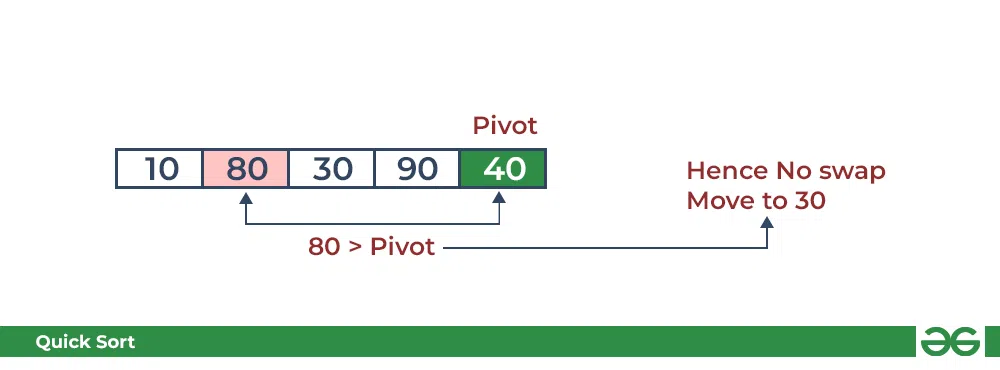Partition in QuickSort: Compare pivot with 80

• Compare 30 with pivot. It is less than pivot so arrange it accordingly.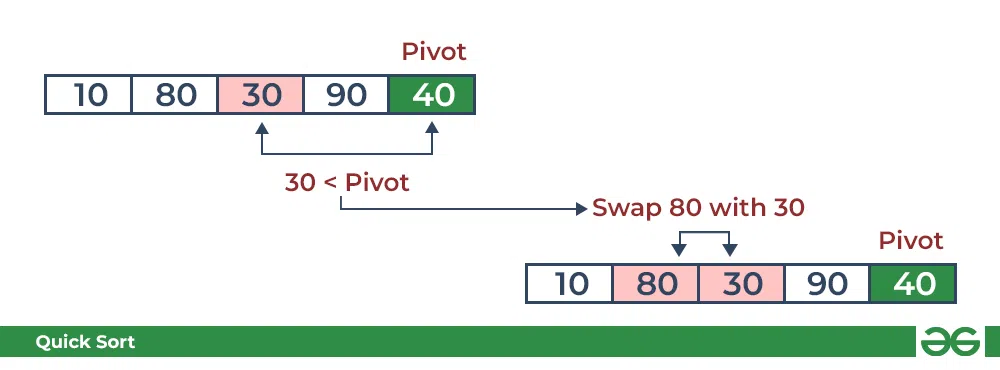Partition in QuickSort: Compare pivot with 30

• Compare 90 with the pivot. It is greater than the pivot.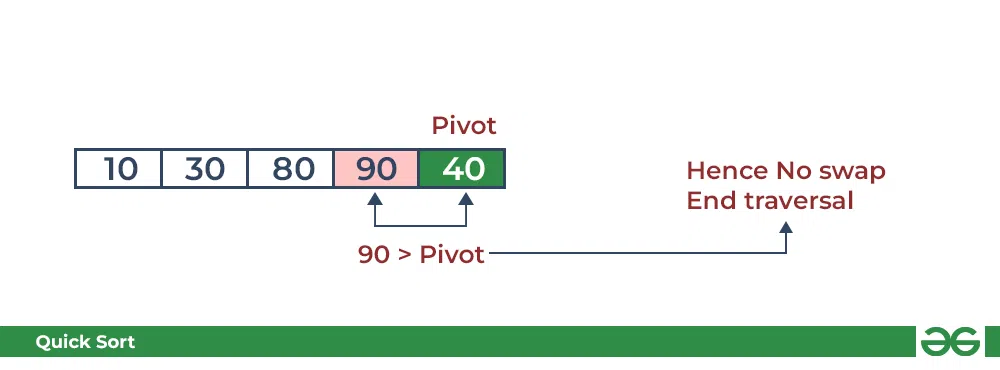Partition in QuickSort: Compare pivot with 90

• Arrange the pivot in its correct position.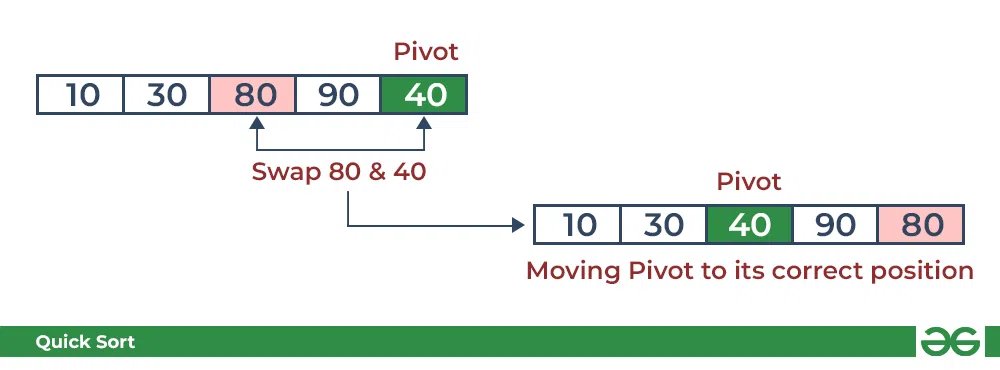Partition in QuickSort: Place pivot in its correct position

### Illustration of Quicksort:

As the partition process is done recursively, it keeps on putting the pivot in its actual position in the sorted array. Repeatedly putting pivots in their actual position makes the array sorted.

Follow the below images to understand how the recursive implementation of the partition algorithm helps to sort the array.

• Initial partition on the main array: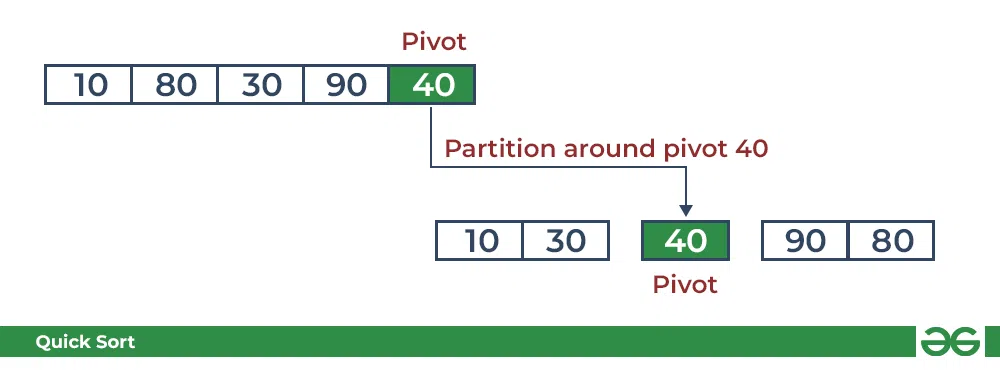Quicksort: Performing the partition

• Partitioning of the subarrays: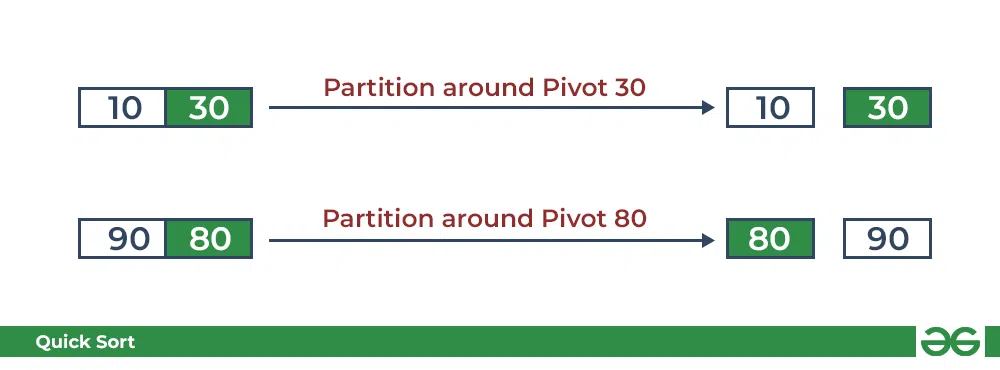Quicksort: Performing the partition

## C

 `// C code to implement quicksort` `#include ` `// Function to swap two elements``void` `swap(``int``* a, ``int``* b)``{``    ``int` `t = *a;``    ``*a = *b;``    ``*b = t;``}` `// Partition the array using the last element as the pivot``int` `partition(``int` `arr[], ``int` `low, ``int` `high)``{``    ``// Choosing the pivot``    ``int` `pivot = arr[high];` `    ``// Index of smaller element and indicates``    ``// the right position of pivot found so far``    ``int` `i = (low - 1);` `    ``for` `(``int` `j = low; j <= high - 1; j++) {` `        ``// If current element is smaller than the pivot``        ``if` `(arr[j] < pivot) {` `            ``// Increment index of smaller element``            ``i++;``            ``swap(&arr[i], &arr[j]);``        ``}``    ``}``    ``swap(&arr[i + 1], &arr[high]);``    ``return` `(i + 1);``}` `// The main function that implements QuickSort``// arr[] --> Array to be sorted,``// low --> Starting index,``// high --> Ending index``void` `quickSort(``int` `arr[], ``int` `low, ``int` `high)``{``    ``if` `(low < high) {` `        ``// pi is partitioning index, arr[p]``        ``// is now at right place``        ``int` `pi = partition(arr, low, high);` `        ``// Separately sort elements before``        ``// partition and after partition``        ``quickSort(arr, low, pi - 1);``        ``quickSort(arr, pi + 1, high);``    ``}``}` `// Driver code``int` `main()``{``    ``int` `arr[] = { 10, 7, 8, 9, 1, 5 };``    ``int` `N = ``sizeof``(arr) / ``sizeof``(arr);` `    ``// Function call``    ``quickSort(arr, 0, N - 1);``    ``printf``(``"Sorted array: \n"``);``    ``for` `(``int` `i = 0; i < N; i++)``        ``printf``(``"%d "``, arr[i]);``    ``return` `0;``}`

## Java

 `// Java implementation of QuickSort``import` `java.io.*;` `class` `GFG {` `    ``// A utility function to swap two elements``    ``static` `void` `swap(``int``[] arr, ``int` `i, ``int` `j)``    ``{``        ``int` `temp = arr[i];``        ``arr[i] = arr[j];``        ``arr[j] = temp;``    ``}` `    ``// This function takes last element as pivot,``    ``// places the pivot element at its correct position``    ``// in sorted array, and places all smaller to left``    ``// of pivot and all greater elements to right of pivot``    ``static` `int` `partition(``int``[] arr, ``int` `low, ``int` `high)``    ``{``        ``// Choosing the pivot``        ``int` `pivot = arr[high];` `        ``// Index of smaller element and indicates``        ``// the right position of pivot found so far``        ``int` `i = (low - ``1``);` `        ``for` `(``int` `j = low; j <= high - ``1``; j++) {` `            ``// If current element is smaller than the pivot``            ``if` `(arr[j] < pivot) {` `                ``// Increment index of smaller element``                ``i++;``                ``swap(arr, i, j);``            ``}``        ``}``        ``swap(arr, i + ``1``, high);``        ``return` `(i + ``1``);``    ``}` `    ``// The main function that implements QuickSort``    ``// arr[] --> Array to be sorted,``    ``// low --> Starting index,``    ``// high --> Ending index``    ``static` `void` `quickSort(``int``[] arr, ``int` `low, ``int` `high)``    ``{``        ``if` `(low < high) {` `            ``// pi is partitioning index, arr[p]``            ``// is now at right place``            ``int` `pi = partition(arr, low, high);` `            ``// Separately sort elements before``            ``// partition and after partition``            ``quickSort(arr, low, pi - ``1``);``            ``quickSort(arr, pi + ``1``, high);``        ``}``    ``}``    ``// To print sorted array``    ``public` `static` `void` `printArr(``int``[] arr)``    ``{``        ``for` `(``int` `i = ``0``; i < arr.length; i++) {``            ``System.out.print(arr[i] + ``" "``);``        ``}``    ``}` `    ``// Driver Code``    ``public` `static` `void` `main(String[] args)``    ``{``        ``int``[] arr = { ``10``, ``7``, ``8``, ``9``, ``1``, ``5` `};``        ``int` `N = arr.length;` `        ``// Function call``        ``quickSort(arr, ``0``, N - ``1``);``        ``System.out.println(``"Sorted array:"``);``        ``printArr(arr);``    ``}``}` `// This code is contributed by Ayush Choudhary``// Improved by Ajay Virmoti`

## Python3

 `# Python3 implementation of QuickSort`  `# Function to find the partition position``def` `partition(array, low, high):` `    ``# Choose the rightmost element as pivot``    ``pivot ``=` `array[high]` `    ``# Pointer for greater element``    ``i ``=` `low ``-` `1` `    ``# Traverse through all elements``    ``# compare each element with pivot``    ``for` `j ``in` `range``(low, high):``        ``if` `array[j] <``=` `pivot:` `            ``# If element smaller than pivot is found``            ``# swap it with the greater element pointed by i``            ``i ``=` `i ``+` `1` `            ``# Swapping element at i with element at j``            ``(array[i], array[j]) ``=` `(array[j], array[i])` `    ``# Swap the pivot element with``    ``# the greater element specified by i``    ``(array[i ``+` `1``], array[high]) ``=` `(array[high], array[i ``+` `1``])` `    ``# Return the position from where partition is done``    ``return` `i ``+` `1`  `# Function to perform quicksort``def` `quicksort(array, low, high):``    ``if` `low < high:` `        ``# Find pivot element such that``        ``# element smaller than pivot are on the left``        ``# element greater than pivot are on the right``        ``pi ``=` `partition(array, low, high)` `        ``# Recursive call on the left of pivot``        ``quicksort(array, low, pi ``-` `1``)` `        ``# Recursive call on the right of pivot``        ``quicksort(array, pi ``+` `1``, high)`  `# Driver code``if` `__name__ ``=``=` `'__main__'``:``    ``array ``=` `[``10``, ``7``, ``8``, ``9``, ``1``, ``5``]``    ``N ``=` `len``(array)` `    ``# Function call``    ``quicksort(array, ``0``, N ``-` `1``)``    ``print``(``'Sorted array:'``)``    ``for` `x ``in` `array:``        ``print``(x, end``=``" "``)` `# This code is contributed by Adnan Aliakbar`

## C#

 `// C# implementation of QuickSort` `using` `System;` `class` `GFG {` `    ``// A utility function to swap two elements``    ``static` `void` `swap(``int``[] arr, ``int` `i, ``int` `j)``    ``{``        ``int` `temp = arr[i];``        ``arr[i] = arr[j];``        ``arr[j] = temp;``    ``}` `    ``// This function takes last element as pivot,``    ``// places the pivot element at its correct position``    ``// in sorted array, and places all smaller to left``    ``// of pivot and all greater elements to right of pivot``    ``static` `int` `partition(``int``[] arr, ``int` `low, ``int` `high)``    ``{``        ``// Choosing the pivot``        ``int` `pivot = arr[high];` `        ``// Index of smaller element and indicates``        ``// the right position of pivot found so far``        ``int` `i = (low - 1);` `        ``for` `(``int` `j = low; j <= high - 1; j++) {` `            ``// If current element is smaller than the pivot``            ``if` `(arr[j] < pivot) {` `                ``// Increment index of smaller element``                ``i++;``                ``swap(arr, i, j);``            ``}``        ``}``        ``swap(arr, i + 1, high);``        ``return` `(i + 1);``    ``}` `    ``// The main function that implements QuickSort``    ``// arr[] --> Array to be sorted,``    ``// low --> Starting index,``    ``// high --> Ending index``    ``static` `void` `quickSort(``int``[] arr, ``int` `low, ``int` `high)``    ``{``        ``if` `(low < high) {` `            ``// pi is partitioning index, arr[p]``            ``// is now at right place``            ``int` `pi = partition(arr, low, high);` `            ``// Separately sort elements before``            ``// and after partition index``            ``quickSort(arr, low, pi - 1);``            ``quickSort(arr, pi + 1, high);``        ``}``    ``}` `    ``// Driver Code``    ``public` `static` `void` `Main()``    ``{``        ``int``[] arr = { 10, 7, 8, 9, 1, 5 };``        ``int` `N = arr.Length;` `        ``// Function call``        ``quickSort(arr, 0, N - 1);``        ``Console.WriteLine(``"Sorted array:"``);``        ``for` `(``int` `i = 0; i < N; i++)``            ``Console.Write(arr[i] + ``" "``);``    ``}``}` `// This code is contributed by gfgking`

## Javascript

 `// Function to partition the array and return the partition index``function` `partition(arr, low, high) {``    ``// Choosing the pivot``    ``let pivot = arr[high];``  ` `    ``// Index of smaller element and indicates the right position of pivot found so far``    ``let i = low - 1;``  ` `    ``for` `(let j = low; j <= high - 1; j++) {``        ``// If current element is smaller than the pivot``        ``if` `(arr[j] < pivot) {``            ``// Increment index of smaller element``            ``i++;``            ``[arr[i], arr[j]] = [arr[j], arr[i]]; ``// Swap elements``        ``}``    ``}``  ` `    ``[arr[i + 1], arr[high]] = [arr[high], arr[i + 1]]; ``// Swap pivot to its correct position``    ``return` `i + 1; ``// Return the partition index``}` `// The main function that implements QuickSort``function` `quickSort(arr, low, high) {``    ``if` `(low < high) {``        ``// pi is the partitioning index, arr[pi] is now at the right place``        ``let pi = partition(arr, low, high);``  ` `        ``// Separately sort elements before partition and after partition``        ``quickSort(arr, low, pi - 1);``        ``quickSort(arr, pi + 1, high);``    ``}``}` `// Driver code``let arr = [10, 7, 8, 9, 1, 5];``let N = arr.length;` `// Function call``quickSort(arr, 0, N - 1);``console.log(``"Sorted array:"``);``console.log(arr.join(``" "``));`

## PHP

 ``

Output

```Sorted array:
1 5 7 8 9 10
```

### Complexity Analysis of Quick Sort:

Time Complexity:

• Best Case: Ω (N log (N))
The best-case scenario for quicksort occur when the pivot chosen at the each step divides the array into roughly equal halves.
In this case, the algorithm will make balanced partitions, leading to efficient Sorting.
• Average Case: θ ( N log (N))
Quicksort’s average-case performance is usually very good in practice, making it one of the fastest sorting Algorithm.
• Worst Case: O(N2)
The worst-case Scenario for Quicksort occur when the pivot at each step consistently results in highly unbalanced partitions. When the array is already sorted and the pivot is always chosen as the smallest or largest element. To mitigate the worst-case Scenario, various techniques are used such as choosing a good pivot (e.g., median of three) and using Randomized algorithm (Randomized Quicksort ) to shuffle the element before sorting.
• Auxiliary Space: O(1), if we don’t consider the recursive stack space. If we consider the recursive stack space then, in the worst case quicksort could make O(N).